# Lossy Multiconductor Transmission Line Circuit

This example shows how to analyze a lossy multiconductor transmission line circuit. The transmission lines are modeled using RLGC distributed elements which are used in signal integrity analysis for accurately capturing high-speed interconnect effects. You can analyze this circuit using RF Toolbox™ `pwlresp` function or using RF Blockset™ Circuit Envelope model. In this example you first compute the time-domain response of the circuit excited by a periodic pulse signal and then you compare the result to a circuit simulated using the RF Blockset Circuit Envelope simulation.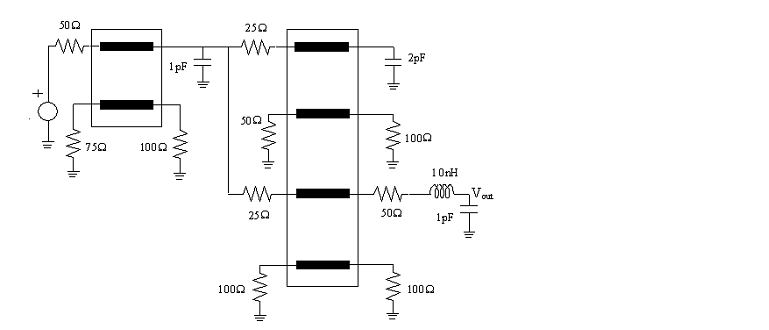Figure 1: Interconnect circuit with lossy multiconductor transmission lines

### Representation of Distributed Transmission Line Models with N-Port Elements

The circuit in this example contains two sets of lossy coupled transmission lines. The RLGC line parameter matrices of the two lines are as follows .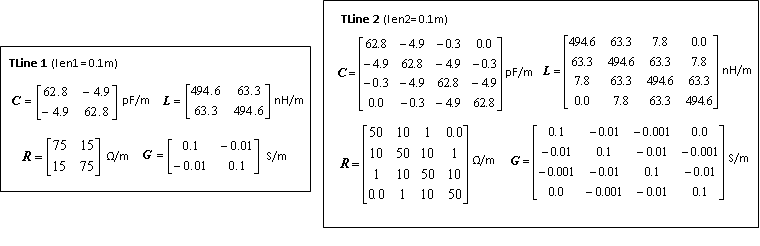Figure 2: RLGC line parameters for the 4-ports and 8-ports transmission lines

Extract the S-parameters of each coupled transmission lines using `rlgc2s` function. Then represent the S-parameters using an `nport` element.

```freq = linspace(1e1,8e9,1001); N = length(freq); len1 = 0.1; R1 = ones(2,2,N).*[75,15;15,75]; L1 = ones(2,2,N).*[494.6,63.3;63.3,494.6]*1e-9; G1 = ones(2,2,N).*[0.1,-0.01;-0.01,0.1]; C1 = ones(2,2,N).*[62.8,-4.9;-4.9,62.8]*1e-12; s_params1 = rlgc2s(R1,L1,G1,C1,len1,freq); stlobj1 = sparameters(s_params1,freq); nport_s1 = nport(stlobj1); len2 = 0.1; R2 = ones(4,4,N).*[50,10,1,0.0;10,50,10,1;1,10,50,10;0.0,1,10,50]; L2 = ones(4,4,N).*[494.6,63.3,7.8,0.0;63.3,494.6,63.3,7.8;7.8,63.3,494.6,63.3;0.0,7.8,63.3,494.6]*1e-9; G2 = ones(4,4,N).*[0.1,-0.01,-0.001,0.0;-0.01,0.1,-0.01,-0.001;-0.001,-0.01,0.1,-0.01;0.0,-0.001,-0.01,0.1]; C2 = ones(4,4,N).*[62.8,-4.9,-0.3,0.0;-4.9,62.8,-4.9,-0.3;-0.3,-4.9,62.8,-4.9;0.0,-0.3,-4.9,62.8]*1e-12; s_params2 = rlgc2s(R2,L2,G2,C2,len2,freq); stlobj2 = sparameters(s_params2,freq); nport_s2 = nport(stlobj2); ```

### Calculation of S-Parameter Object of Interconnect Circuit

Calculate the S-parameter object of the circuit given in Figure.1 by using the `sparameters` function.

```ckt = circuit('interconnect'); add(ckt,[1 2],resistor(50)) add(ckt,[2 3 4 5],nport_s1,{'p1+' 'p2+' 'p3+' 'p4+'}) add(ckt,[3 0],resistor(75)) add(ckt,[4 0],capacitor(1e-12)) add(ckt,[5 0],resistor(100)) add(ckt,[4 6],resistor(25)) add(ckt,[7 0],resistor(50)) add(ckt,[4 8],resistor(25)) add(ckt,[9 0],resistor(100)) add(ckt,[6 7 8 9 10 11 12 13],nport_s2,{'p1+' 'p2+' 'p3+' 'p4+' 'p5+' 'p6+' 'p7+' 'p8+'}) add(ckt,[10 0],capacitor(2e-12)) add(ckt,[11 0],resistor(100)) add(ckt,[13 0],resistor(100)) add(ckt,[12 14],resistor(50)) add(ckt,[14 15],inductor(10e-9)) add(ckt,[15 0],capacitor(1e-12)) freqfit = linspace(1e1,8e9,1001)'; setports(ckt,[1 0],[15 0]) S_vout = sparameters(ckt,freqfit); ```

### Generation of Rational Object of Multiconductor Transmission Line Circuit

Convert the transfer function of the S-parameter object of the circuit to a `rational` object over the specified frequencies, . Then calculate the frequency response using the `freqresp` function.

```tfblkout = s2tf(S_vout,0,Inf,2); fitblkout = rational(freqfit,tfblkout); plot(freqfit/1e9,abs(tfblkout),'b',freqfit/1e9,abs(freqresp(fitblkout,freqfit)),'r:','LineWidth',2) legend('Original data','Fitting result') title('Frequency Response') ylabel('Magnitude') xlabel('Frequency (GHz)') ```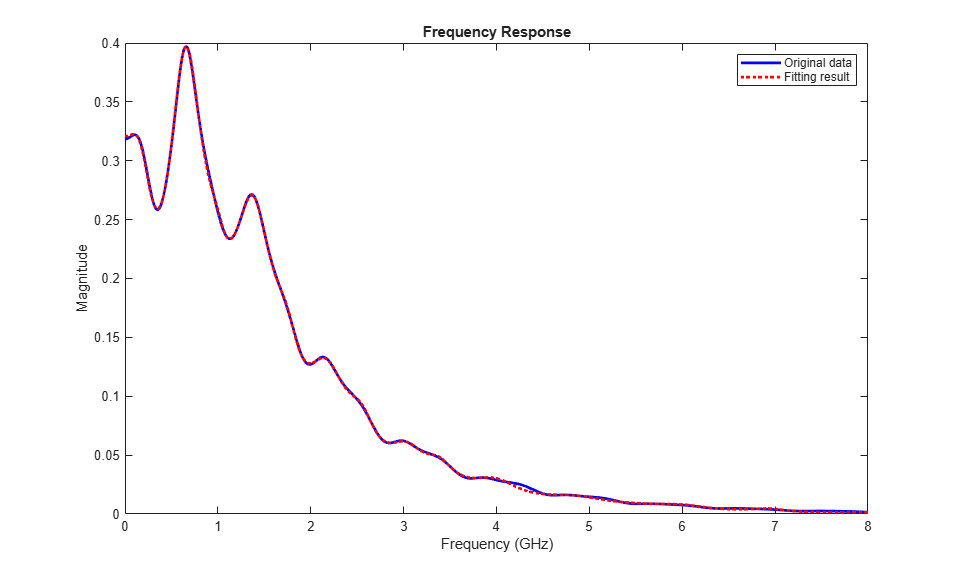### Computation of Transient Waveform Excited by Periodic Pulse Source

Apply a voltage source using a 1 V periodic pulse with a rise/fall time of 0.4 ns, a duration of 5 ns, and a period of 1.6 ns. Then compute the time-domain response of the circuit by using the `pwlresp` function.

```SignalTime = [0,0.4,5.4,5.8]*1e-9; SignalValue = [0,1,1,0]; Tsim = (0:1e-11:6e-8); TP = 1.6e-8; [WAVEOUT, tout] = pwlresp(fitblkout,SignalTime,SignalValue,Tsim,TP); plot(tout,WAVEOUT, 'LineWidth',2) legend('PWLRESP') title('Output Waveform') xlabel('Time (s)') ylabel('Output (V)') ylim([-0.05 0.38]) ```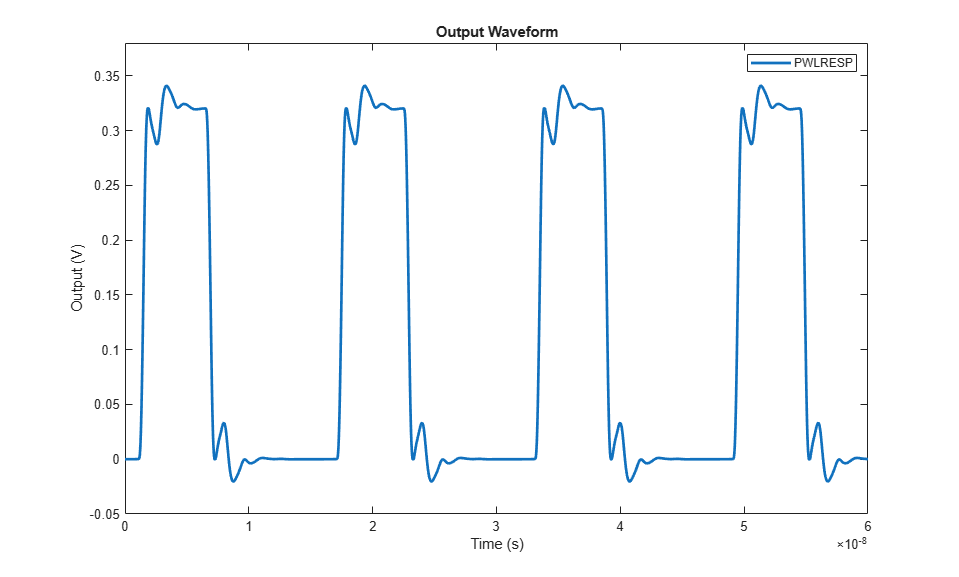### Comparison of Time-Domain Response of Coupled Transmission Line Circuit Using RF Blockset

A Simulink model is built to simulate the multiconductor transmission line circuit shown in Figure 1. With the S-parameters calculated from previous session, the multiconductor transmission lines can be represented by S-parameter blocks from RF Blockset Circuit Envelope Library. For instance, the 4-port transmission lines are modeled by defining the S-parameter block mask as shown below, where `STL4p` is a S-parameter object converted from reordering ports of S-parameter object `stlobj1` with `snp2smp` function. The output of the model is the time-domain response in the form of a passband signal. For more information on passband signal implementation in the Circuit Envelope model, see Passband Signal Representation in Circuit Envelope (RF Blockset).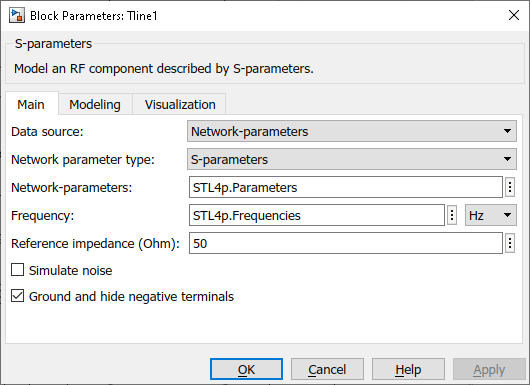To simulate the model:

1. Type `open_system('simrf_coupled_pb')` at the command prompt.

2. Select Simulation > Run.

After you finish simulating the model, compare the obtained transient waveform with that calculated using RF Toolbox.

```open_system('simrf_coupled_pb'); sim('simrf_coupled_pb'); plot(tout,WAVEOUT,CE_Data(:,1),CE_Data(:,2),'--','LineWidth',2) legend('PWLRESP', 'Circuit Envelope') title('Time-Domain Response of Lossy Coupled Transmission Lines') xlabel('Time (s)') ylabel('Output (V)') ylim([-0.05 0.38]) ```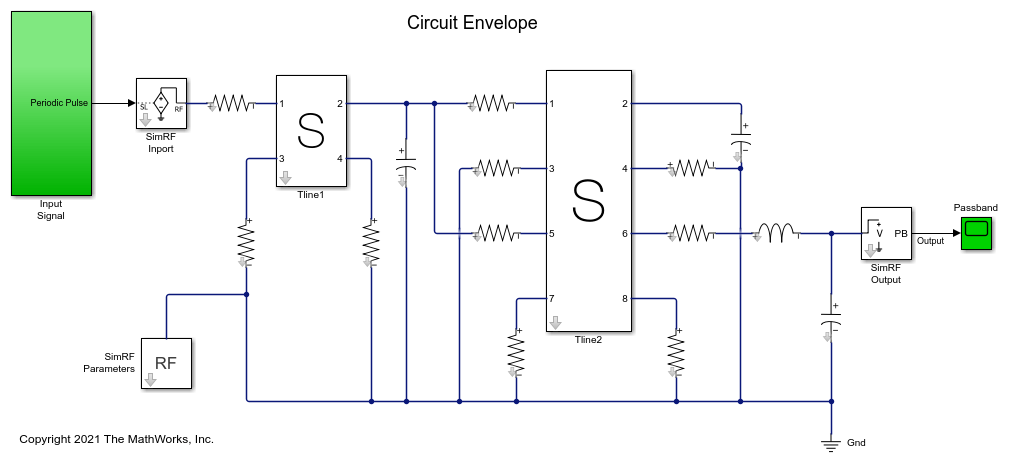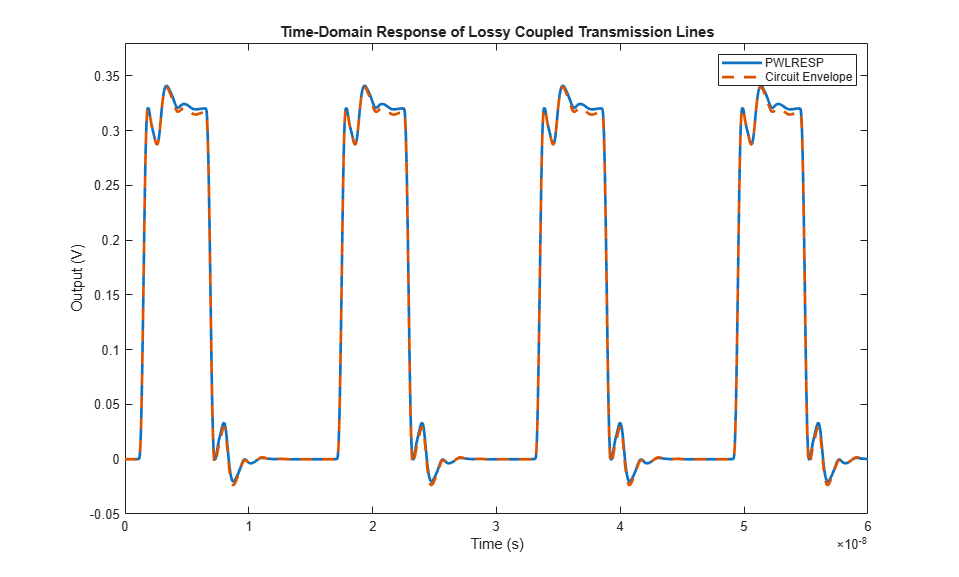```bdclose('simrf_coupled_pb'); ```

 Tang, Tak K., and Michel Nakhla. “Analysis of High-Speed VLSI Interconnects Using the Asymptotic Waveform Evaluation Technique.” IEEE Transactions on Computer-Aided Design of Integrated Circuits and Systems 11, no. 3 (March 1992): 341–52. https://doi.org/10.1109/43.124421.